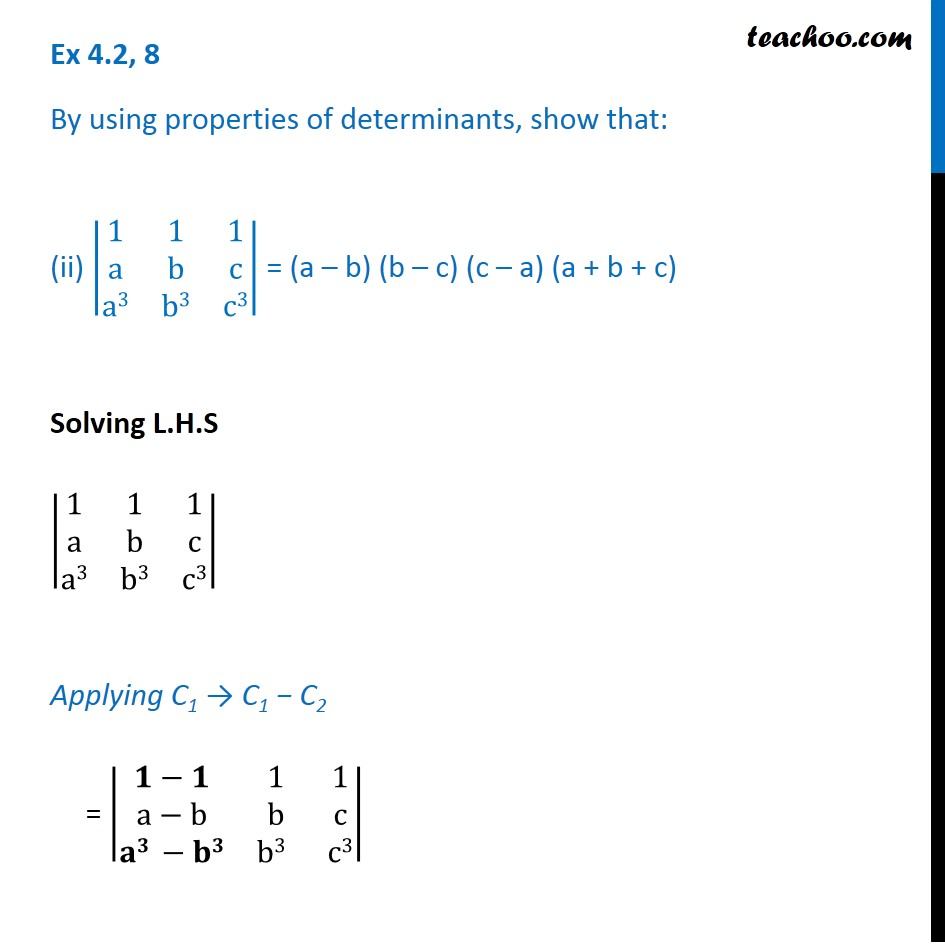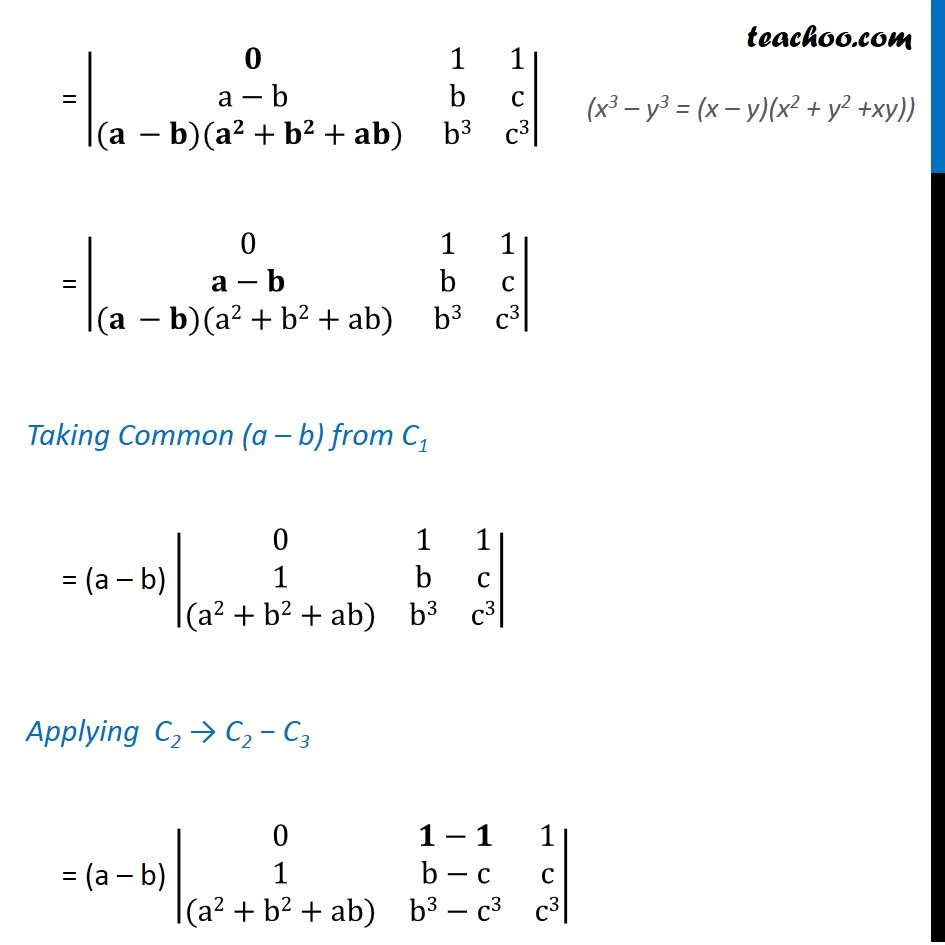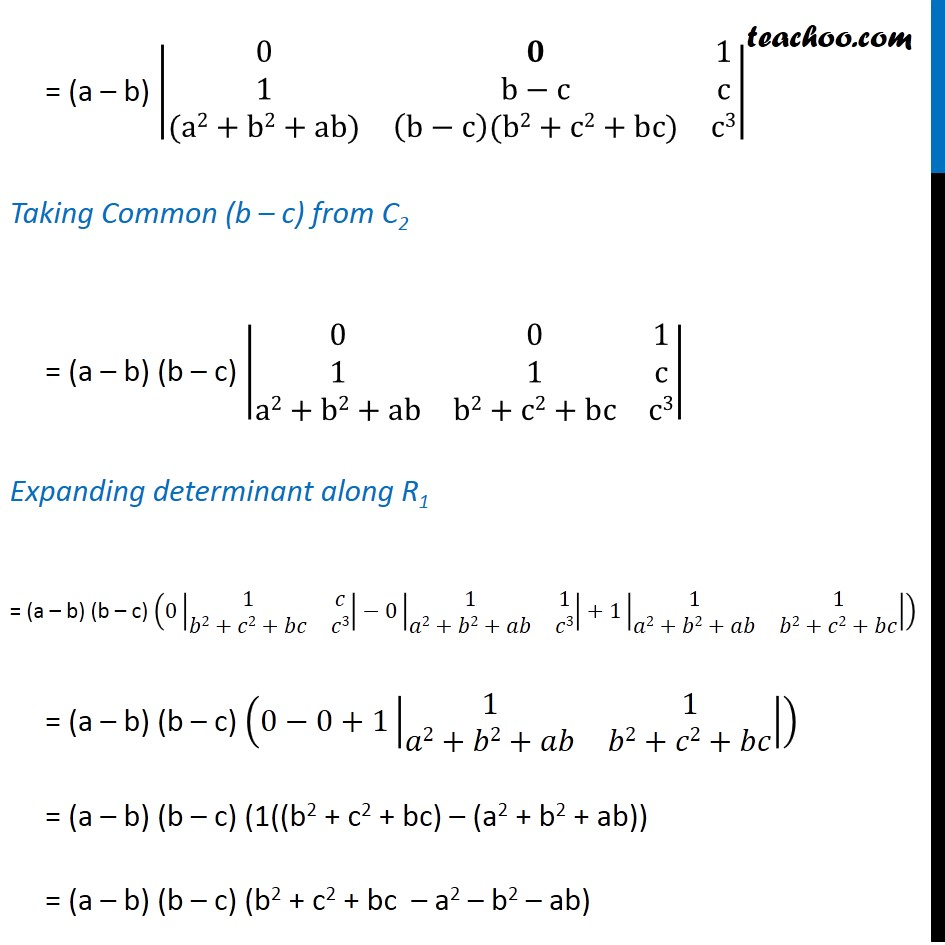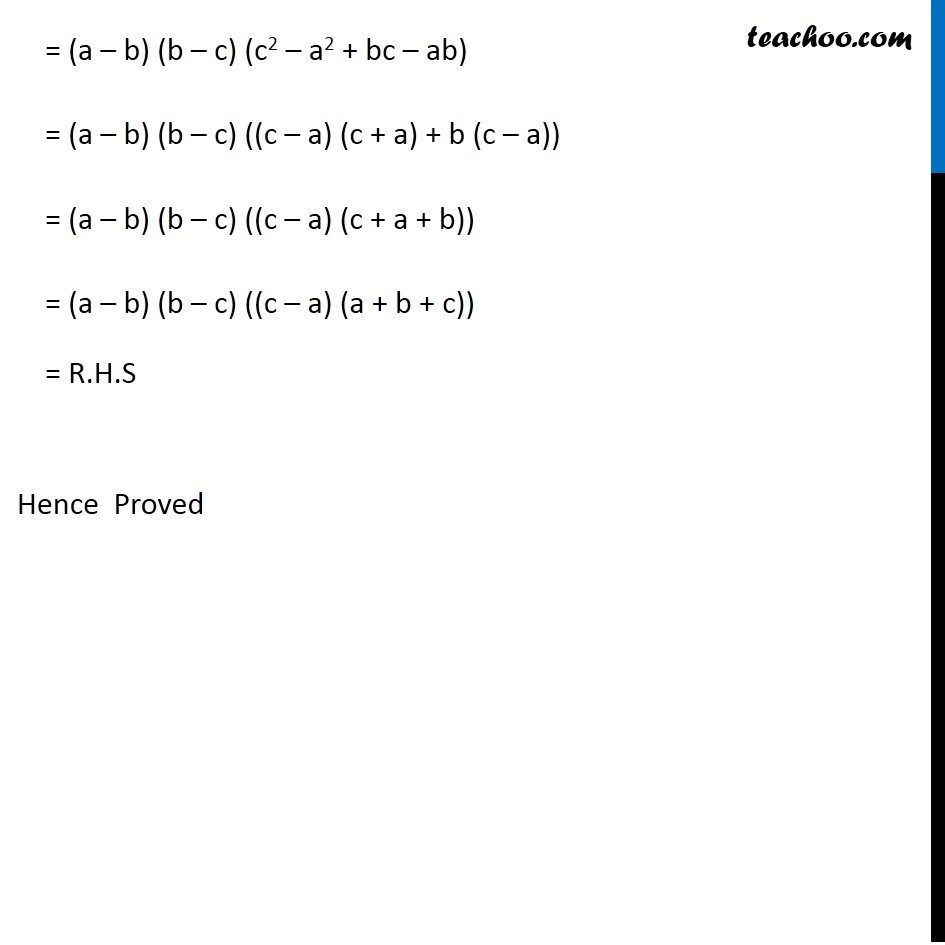Properties of Determinant

Chapter 4 Class 12 Determinants
Serial order wiseLearn in your speed, with individual attention - Teachoo Maths 1-on-1 Class

### Transcript

Question 8 By using properties of determinants, show that: (ii) |■8(1&1&[email protected]&b&[email protected]&b3&c3)| = (a – b) (b – c) (c – a) (a + b + c) Solving L.H.S |■8(1&1&[email protected]&b&[email protected]&b3&c3)| Applying C1 → C1 − C2 = |■8(𝟏−𝟏&1&[email protected]−b&b&c@𝐚𝟑 −𝐛𝟑&b3 &c3)| = |■8(𝟎&1&[email protected]−b&b&c@(𝐚 −𝐛)(𝐚𝟐+𝐛𝟐+𝐚𝐛) &b3&c3)| = |■8(0&1&1@𝐚−𝐛&b&c@(𝐚 −𝐛)(a2+b2+ab) &b3&c3)| Taking Common (a – b) from C1 = (a – b) |■8(0&1&[email protected]&b&c@(a2+b2+ab)&b3&c3)| Applying C2 → C2 − C3 = (a – b) |■8(0&𝟏−𝟏&[email protected]&b−c&c@(a2+b2+ab)&b3−c3&c3)| (x3 – y3 = (x – y)(x2 + y2 +xy)) = (a – b) |■8(0&𝟎&[email protected]&b−c&c@(a2+b2+ab)&(b−c)(b2+c2+bc)&c3)| Taking Common (b – c) from C2 = (a – b) (b – c) |■8(0&0&[email protected]&1&[email protected]+b2+ab&b2+c2+bc&c3)| Expanding determinant along R1 = (a – b) (b – c) (0|■8(1&𝑐@𝑏2+𝑐2+𝑏𝑐&𝑐3)|−0|■8(1&1@𝑎2+𝑏2+𝑎𝑏&𝑐3)|+1|■8(1&1@𝑎2+𝑏2+𝑎𝑏&𝑏2+𝑐2+𝑏𝑐)|) = (a – b) (b – c) (0−0+1|■8(1&1@𝑎2+𝑏2+𝑎𝑏&𝑏2+𝑐2+𝑏𝑐)|) = (a – b) (b – c) (1((b2 + c2 + bc) – (a2 + b2 + ab)) = (a – b) (b – c) (b2 + c2 + bc – a2 – b2 – ab) = (a – b) (b – c) (c2 – a2 + bc – ab) = (a – b) (b – c) ((c – a) (c + a) + b (c – a)) = (a – b) (b – c) ((c – a) (c + a + b)) = (a – b) (b – c) ((c – a) (a + b + c)) = R.H.S Hence Proved